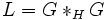# Normal not implies amalgam-characteristic

Jump to: navigation, search
This article gives the statement and possibly, proof, of a non-implication relation between two subgroup properties. That is, it states that every subgroup satisfying the first subgroup property (i.e., normal subgroup) need not satisfy the second subgroup property (i.e., amalgam-characteristic subgroup)
View a complete list of subgroup property non-implications | View a complete list of subgroup property implications
Get more facts about normal subgroup|Get more facts about amalgam-characteristic subgroup
EXPLORE EXAMPLES YOURSELF: View examples of subgroups satisfying property normal subgroup but not amalgam-characteristic subgroup|View examples of subgroups satisfying property normal subgroup and amalgam-characteristic subgroup

## Statement

### Verbal statement

A normal subgroup of a group need not be an amalgam-characteristic subgroup.

### Statement with symbols

Let$G$ be a group and$H$ be a normal subgroup of$G$. Let$L = G *_H G$. Then, it is not necessary that$H$ is characteristic in$L$.

## Proof

### Example of the free group

Let$F$ be a free group on two generators and$\mathbb{Z}$ be the group of integers. Let$G = F \times \mathbb{Z}$ and$H = F \times \{ 0 \}$ be the embedded first direct factor. We have:$L = (F \times \mathbb{Z}) *_{F \times \{ 0 \}} (F \times \mathbb{Z}) = F \times (\mathbb{Z} * \mathbb{Z}) \cong F \times F$.

Thus,$L$ is a direct product of two copies of the free group on two generators, and moreover, the embedded subgroup$H$ in$L$ is simply$F \times \{ e \}$, the first embedded direct factor. This is not a characteristic subgroup in$L$, because there exists an exchange automorphism swapping the two direct factors of$L$.